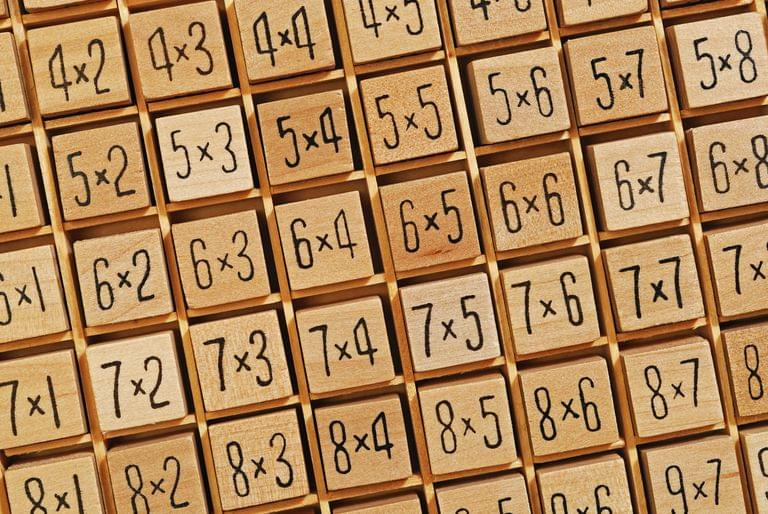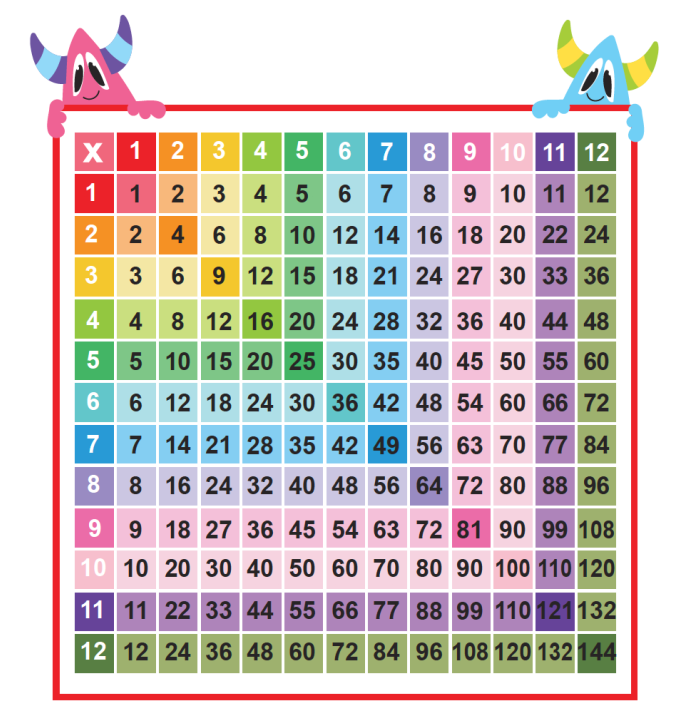# MultiplicationChartHelping children master multiplication charts can be a daunting task for parents, teachers and even the children. The thought that they have to memorize the entire table to succeed in math can be scary for kids, especially those struggling with number work.

Whether you are a parent trying to help your child study at home, or a student looking for ways to master your multiplication table, starting from the basics and following through step by step is the key to a good mathematical foundation.

What are these step by step strategies and are they the best ways to learn multiplication charts?

Well, let's dig in to find out.

This article explains how to learn multiplication using pictures and charts. It further provides steps to consider when learning it.

### What is multiplication?

Multiplication is calculating the total of a number multiplied by another.

It is also considered a repeated addition because it shares the same concepts in arriving at an answer.

Multiplication is also a way of adding equal groups of numbers.

Because every school child at some point must learn and perfect the multiplication chart, they must know the steps to follow.

When working on perfecting the multiplication tables, consider knowing the fundamentals first.

This makes it easy to manipulate bigger numbers and memorize the table.

Rote learning isn't the best way to make it stick.

What's the best way? Consider these 6 step by step tips.

#### 1. Perfect your skip counting:

By first and second grades pupils are introduced to skip counting. Being able to perfect skip counting from 2 through to 10, sets a solid ground for multiplication charts. They are both linked.

Skip counting is counting forward. If you observe you will see that it sets the pattern for the multiplication table.

When skip counting you just keep adding the original number to a new product it gives you. For example, skip counting in 3's. You start with the number 3 and add another 3 to it, which gives you 6, you continue adding 3 until you get the final product you want.

Procedure

3, 6, 9, 12, 15……

When you skip count in 2's note that they are all even numbers. The odd numbers are left out.

Example, 2 -- 4-- 6-- 8--10…….

Skip counting helps you easily find the answer to a multiplication problem and master the time table.

For instance, skip counting in 4's leads you to the answer for the 4-times table.

Procedure

4, 8, 12, 16, 20 is number 4 repeated 5 times which is the same as 4×5= 20, that's

4×1= 4

4×2= 8

4×3= 12

4×4= 16

4×5= 20

There is no way a child will perfect this step in second grade and not find the multiplication table easy.

#### 2. Use Repeated Addition

Repeated addition means adding up equal groups of numbers again and again. It sets the basis for multiplication.

For example, a question that says: 6 groups of 2, or 6 lots of 2, is the same as 2 in 6 places. That is 2 added to itself 6 times.

Procedure

2 + 2 + 2 + 2 + 2 + 2=12

is the same as 2×6 = 12.

Learning this step is a fast way of knowing the concept of the multiplication table.

Note that using repeated addition means that the number of times you add an equal group of numbers is the same as the number you added multiplied by how many times you've added it.

#### 3. Learn the smaller numbers first:

Learning starts from simple to complex.

Whether you're a teacher teaching a pupil, a parent helping your child study at home, or a student learning multiplication table on your own, ensure the smaller numbers are perfected first.

Don't just go learning the 5 or 10 times table because you know they are the easiest. It's best to start from as small as a 1-time table, master it before moving to the next.

Knowing the rules that, 1× any number will result in the same number is very vital. E.g 1×1=1, 1×2=2…..

#### 4. Reinforce the commutative property:

In cumulative property, all you need to do is turn the given numbers to reverse then multiple and you still get the same end product.

For example:

2×3=6 and 3×2=6

3×4=12 and 4×3=12.

Learning this step will help master all of such numbers in the table.

#### 5. Learn these hidden tips:

The eleven times table is just a repeat of the number you are multiplying with that serves as the product.

For example, if you multiply 11×2, just write the 2 again and add another two, it gives you the product.

So,

11×1=11

11×2= 22

11×3= 33…..

It's the same with the 10 times table, just add 0 to the number you are multiplying the 10 with and you get the answer you need.

That's,

10×1= 10

10×2= 20

10×3= 30…

## catch up in school

#### 6. Learn Using Visuals

Everyone learns differently.

Some children will need the aid of visuals to enable them to recall what has been learnt. Including pictures, worksheets and manipulative can serve as a big help.

Use pictures and concrete examples to elaborate when teaching.

### How To Use Visuals?

#### Make Button Arrays

An array is arranging sets of objects into columns and rows.

Objects in columns are arranged from top to bottom while objects in rows, from left to right.

You can get different colored buttons, arrange them according to the times table number you are struggling with, then stick them on cardboard.

When 5×4 is arranged, it will appear as 4 buttons on a row and 5 buttons on a column. Count all buttons together and you get 20 in total. That's 5×4=20

#### Paper Wheel Chart

Many kids struggle with memorizing the 7 and 9 times tables. To make it easy, create a paper wheel chart of each multiplication number a kid is struggling with.

#### Procedure

First, divide the wheel into 3 circles. Let the digit number you're multiplying with be in the middle. The numbers you are multiplying stays in the second part of the circle. And the product you get, on the third part of the circle.

You can draw a line across each number set to make your work appear neat.

#### Create Your Chart

Doing it yourself is the best way to learn. Children need to make their multiplication chart.

It's a task a learner should be taught to commit to.

Decide how many days your child needs to complete their table. Do the rough work and use repeated addition to draw up the chart.

For example, 5+5=10 is 5×2=10

When this rough addition is completed, pupils can start transferring the results to a template to complete their multiplication chart.

With this, it's easy to memorize the chart. Because it's much easier to recall what you did.

#### Practice with worksheets

A worksheet is a helpful resource for kids' practice. They are fully engaging.

Practising multiplication with a worksheet gives pupils the chance to problem-solve and think up answers.

For kids, worksheets are more fun to practice with than regular notebooks.

Teachers or parents can provide the next step of the multiplication worksheet once pupils perfect a table.

You should provide children with access to practice exercise sheets with colourful details that appeal to the senses. Like using worksheets with animated character templates.

Have them colour every correct answer they provide.

### Displaying Pictures and Charts As A Guide

After all the pictures, charts and worksheets that children are exposed to while learning multiplication, it's most important that these visuals are displayed as a reminder and guide for them; in the classroom or at home, in study spots or their bedroom.

The overall message is that seeing these visuals every day makes it easy to memorize the time table. Because they become familiar with it.

### Conclusion

Multiplication is one of the basic parts of mathematics that is needed in everyday life.

It also sets the foundation for further higher math concepts like fractions, among others.

Following the above steps is an effective way of learning the multiplication chart.

Furthermore, when dealing with math, kids have to practice daily. The concept is the same as memorizing the chart.

Dedicating at least 10 to 15 minutes every day (maybe before bed) to reciting the table makes it stick to your memory.